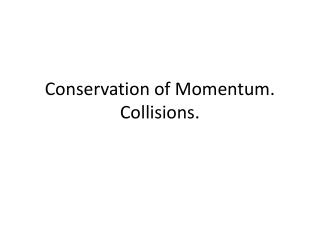Download PresentationConservation of Momentum. Collisions.

# Conservation of Momentum. Collisions.

Download Presentation## Conservation of Momentum. Collisions.

- - - - - - - - - - - - - - - - - - - - - - - - - - - E N D - - - - - - - - - - - - - - - - - - - - - - - - - - -
##### Presentation Transcript

1. Conservation of Momentum. Collisions.

2. Conserved Momentum • An impulse must be exerted on the object by something outside the object to change its momentum. If no outside force is present, no change in momentum is possible. It is an isolated system. • If no net force or net impulse acts on a system, the momentum of that system cannot change. A system will have the same momentum before some internal interaction as it has after the interaction occurs. The momentum that does not change is conserved.

3. The Law of Conservation of Momentum • The Law of Conservation of Momentum: In the absence of an external force, the momentum of a system remains unchanged. The examples: the atomic nuclei undergoing radioactive decay, cars colliding, and stars exploding.

4. Collisions. • The collision of objects shows the conservation of momentum. If objects collide in the absence of external forces, the net momentum of both objects before collision equals the net momentum of both objects after collision. net momentumbefore collision =net momentumafter collision pbefore= pafter

5. Elastic Collisions • When objects collide without being permanently deformed and without generating heat, the collision is called elastic. • Colliding objects bounce perfectly in perfect elastic collision. The sum of the momentums is the same before and after each collision. m1*v1+m2*v2=m1*v1f+m2*v2f elastic collision where m1 and m2 –masses of the colliding objects v1, and v2 - velocities of objects before collision, V1f and v2f – velocities of objects after collision.

6. Elastic Collision

7. Inelastic collision • If colliding objects become distorted, tangled, couple together and generate heat during collision, a inelastic collision occurs. net momentumbefore collision =net momentumafter collision pbefore = pafter • m1*v1+m2*v2=(m1+m2)*v2f inelastic collision where m1 and m2 –masses of the colliding objects v1, and v2 - velocities of objects before collision, v2f – velocity of coupled objects after collision.

8. Inelastic Collision

9. Collisions • Most collisions involve some external force. • The Examples: • Billiard balls encounter friction with the table and the air. The collided combined trucks encounter friction that provides impulse to decrease their momentum. • These external forces are usually negligible during the collision, so the net momentum does not change during collision. • A pair of space vehicles docking in orbit have the same net momentum before and after contact. The combine momentum of the space vehicles after docking changes only by gravity because there is no air resistance.